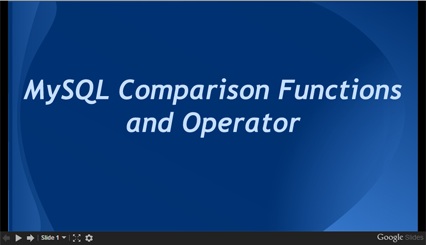# MySQL NULL safe equal to operator

## NULL safe equal to operator

MySQL null safe equal to operator performs an equality comparison like the equal to (=) operator, but returns 1 rather than NULL if both operands are NULL, and 0 rather than NULL if one operand is NULL.

Syntax:

`<=>`

MySQL Version: 5.6

Example: MySQL NULL safe equal to operator

The following MySQL statement compares if 1 is less than, equal to or greater than NULL; if NULL is less than, equal to or greater than NULL and if 3 is less than, equal to or greater than NULL.

Code:

``````SELECT NULL <=> 1, NULL <=> NULL, 3 <=> NULL;
```
```

Sample Output:

```mysql> SELECT NULL <=> 1, NULL <=> NULL, 3 <=> NULL;
+------------+---------------+------------+
| NULL <=> 1 | NULL <=> NULL | 3 <=> NULL |
+------------+---------------+------------+
|          0 |             1 |          0 |
+------------+---------------+------------+
1 row in set (0.03 sec)
```

Slideshow of MySQL Comparison Function and OperatorsPrevious: COALESCE()
Next: Equal operator(=)

﻿